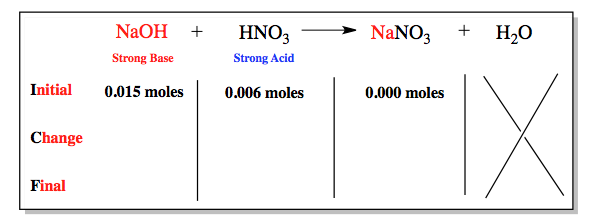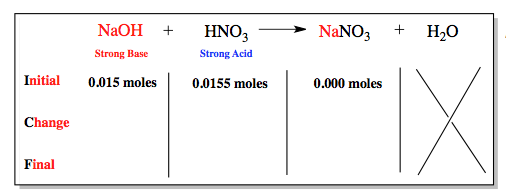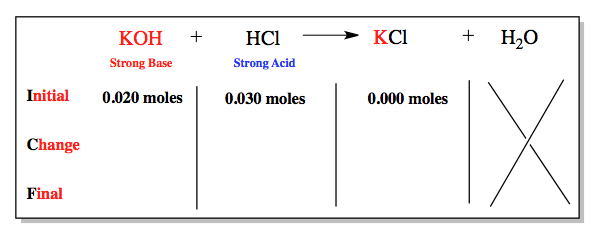Clutch Prep is now a part of Pearson
Ch.10 - Acid-Base TitrationsWorksheetSee all chapters

# Strong Acid-Strong Base Titrations

See all sections
Sections
Strong Acid-Strong Base Titrations
Weak Acid-Strong Base Titrations
Weak Base-Strong Acid Titrations
Diprotic Acid Titrations
Polyprotic Titrations

In a Strong Acid-Strong Base Titration we have the formation of a sigmodial shaped graph.

###### Strong Base-Strong Acid Titration

Concept #1: In these series of titrations the strong base is the starting solution or analyte and the strong acid is the titrant.

Concept #2: At all points before the equivalence point we calculate the amount of strong base remaining through an ICF Chart.Example #1: At the equivalence point for two strong species the pH will equal 7.00

Concept #3: After the equivalence point there will remain an excess of strong acid.Example #2: Calculate the pH of the solution resulting from the titration of 150.0 mL of 0.20 M NaOH with 80.0 mL of 0.15 M HBr.

Example #3: Calculate the pH of the solution resulting from the titration of 100.0 mL of 0.30 M LiH with 150.0 mL of 0.40 M Hl.

###### Strong Acid-Strong Base Titration

Concept #4: In these series of titrations the strong acid is the starting solution or analyte and the strong base is the titrant.

Concept #5: At all points before the equivalence point we calculate the amount of strong acid remaining through an ICF Chart.Concept #6: At the equivalence point for two strong species the pH will equal 7.00

Concept #7: After the equivalence point there will remain an excess of strong base.

Example #4: Calculate the pH of the solution resulting from the titration of 50.0 mL of 0.10 M HI with 20.0 mL of 0.30 M NaOH.

Example #5: Calculate the pH of the solution resulting from the titration of 90.0 mL of 0.40 M HClO3 with 50.0 mL of 0.50 M KOH.